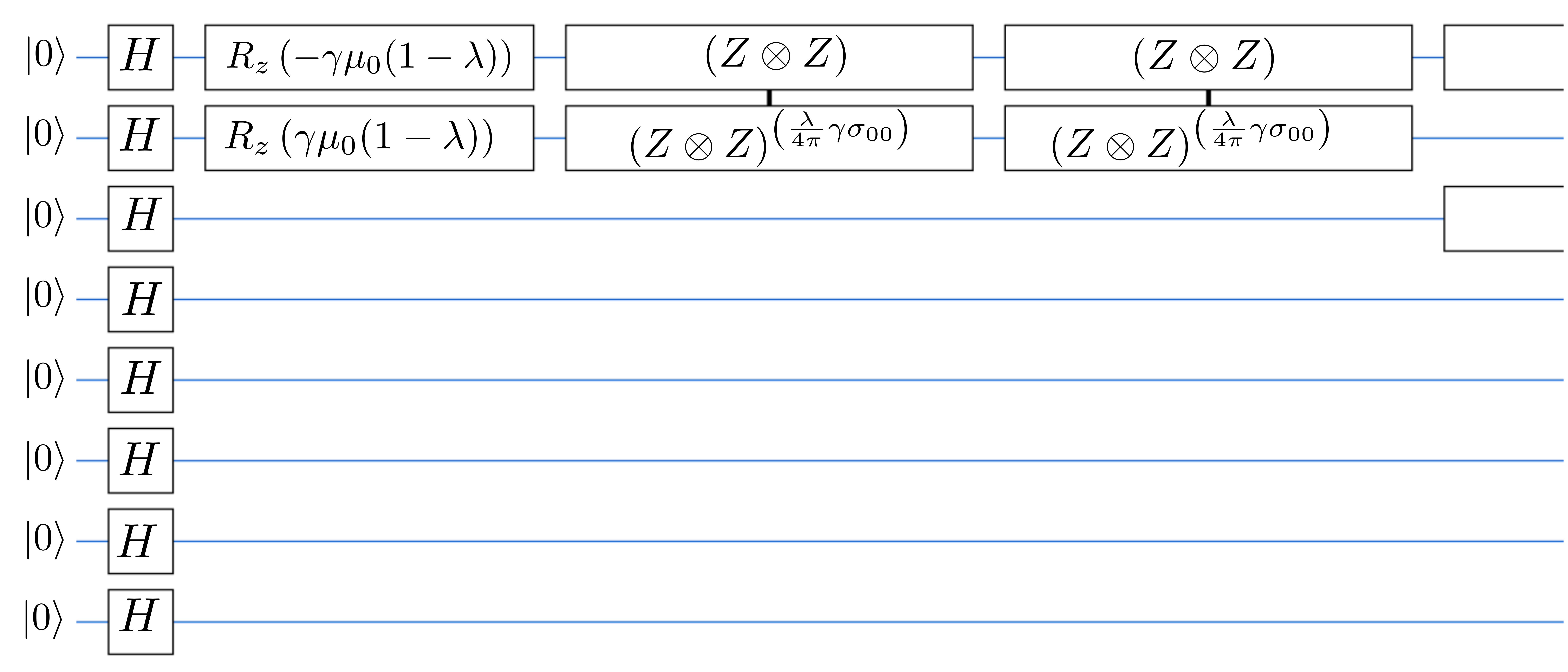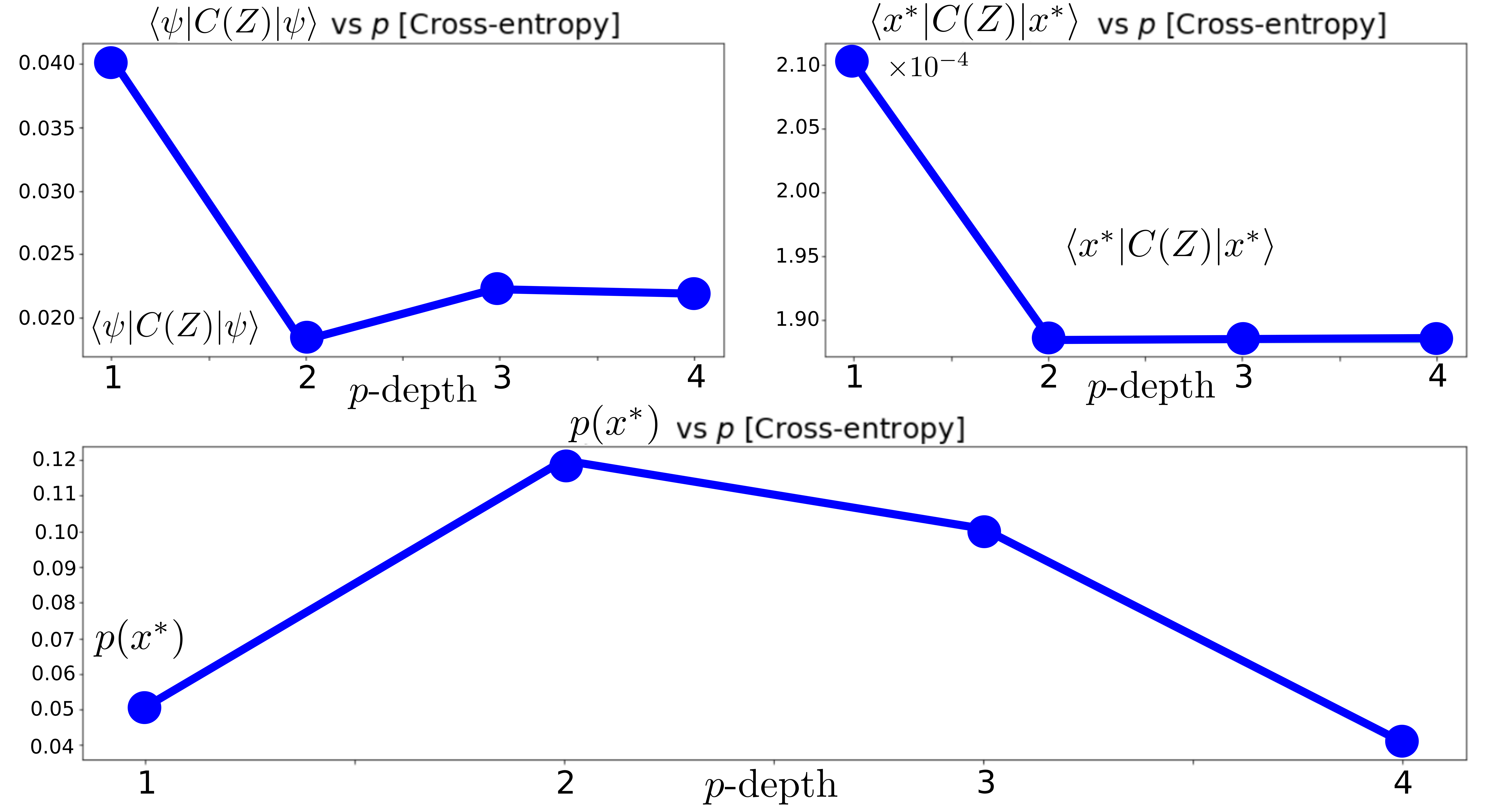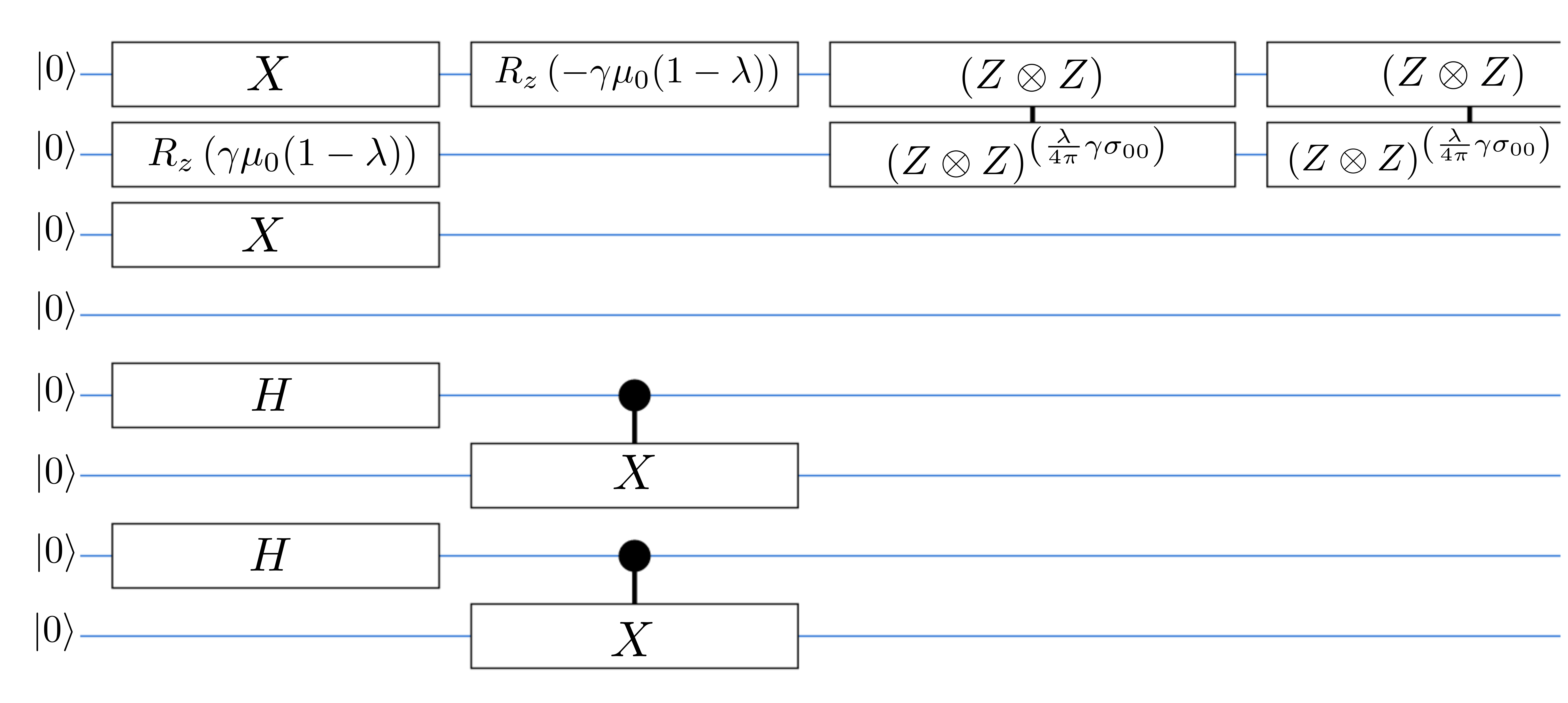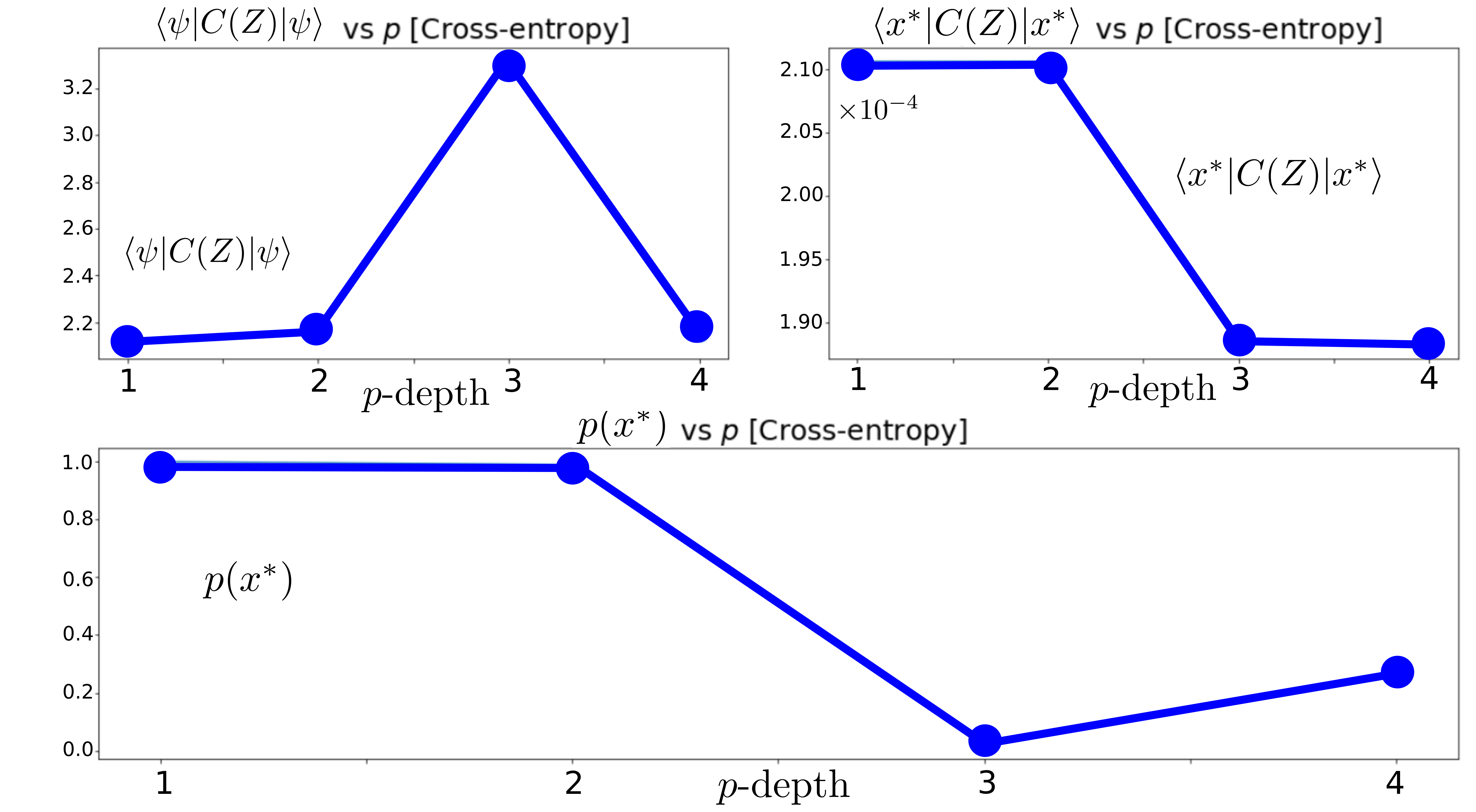# Optimized portfolios with variational quantum algorithms

June 17, 2020

Blog post written by: Oscar Javier Hernandez

###### Quantum computers have the potential to show large speed-ups over their classical counterparts and disrupt industries such as finance in the future. Following the work of M. Hodson et. al [arxiv:1911.05296] in this blog post we implement variational quantum algorithms to solve the financial portfolio optimization problem. Investment constraints are imposed in two different ways, the first method uses a penalization term while the second method constrains the feasible space of solutions.  This work was carried out with assistance from Guoming Wang from Zapata Computing and a mentor from the quantum open source foundation (QOSF). The accompanying Jupyter notebook and source code are available as an open-source project for anyone else to use.

link to the Quantum open source foundation

### ‍Introduction

Given the set of all possible stocks, what is the best way to choose the subset that optimizes financial objectives such as maximizing returns or minimizing the investment risks for an asset manager or individual? This problem is known as the portfolio-optimization problem and is rooted in the work of Harry Markowitz from 1952. In the original formulation, the optimal portfolio allocation is the one that produces the maximum ratio between the portfolio return and its risk. This problem can be stated as an optimization problem where the objective is to minimize the quadratic objective function

$$C({\bf w})= \lambda{\bf w}^T \Sigma {\bf w} -(1-\lambda ){\bf u} \cdot {\bf w},$$

where $\Sigma$ is the $N \times N$ covariance matrix of the $N$-selected stocks , ${\bf w}$ represents the percentage % of invested capital in a given asset (with $\sum_i w_i =1$), ${\bf u}$ is the vector representing the expected returns of the stocks and $0 \leq \lambda \leq 1$ is the asset manager control parameter. The first term ${\bf w}^T \Sigma {\bf w}$ in this expression represents the volatility of the chosen portfolio, while the second term ${\bf u} \cdot {\bf w}$ represents the expected returns. Therefore, a low value of $\lambda$ favors the expected returns of the portfolio while a value approaching $1$ favors low volatility. This simple cost function can be extended to include other effects, such as rebalancing costs and market impact.

In this post, we will focus on solving the discrete version of this problem. In this formulation, instead of the continuous holding vector ${\bf w}$ which represent percentage allocations, we have the discrete holding vector ${\bf z}$ that consists of integer entries where $+1$ is a hold position, $-1$ a short position and $0$ indicates no position. For a set of $N$ stocks, there are $3^N$ feasible portfolio holding combinations to compute and therefore solving the discrete portfolio optimization problem becomes exponentially more difficult with larger $N$. Furthermore, in the discrete version of this problem, we are interested in imposing the constraint

$$\sum_i z_i =D,$$

that represents our desire to have exactly $D$ equally valued positions to be invested in each stock.

Due to the exponentially increasing problem size, this problem can be very slow to solve on a classical computer when $N$ becomes large. For an $N$ -qubit Quantum computer, $2^N$-possible bitstrings can be stored as a quantum state, allowing quantum computers the potential to sample these exponentially growing model spaces and determine the optimal values more efficiently than a classical computer. Currently available quantum hardware exists as noisy intermediate-scale quantum computers (NISQ) available through cloud-based APIS. Quantum algorithms such as the Quantum approximate optimization algorithm (QAOA) are available that can be executed on these NISQ devices as well as on simulators.

The improved speed and solution quality that can be provided by quantum algorithms is very attractive for solving mathematical finance problems. In this post we implement the algorithm presented in arxiv:1911.05296 for solving the discrete portfolio optimization with investment constraints on a universal gate-based quantum computer using two different variational quantum algorithms to handle constraints, run it on a simulator and compare their results.

In the next section we will provide an overview of the two specific algorithms that were used to solve this problem.

### The Quantum approximate optimization algorithm and the Quantum alternating operator ansatz

The quantum approximate optimization algorithm (QAOA) is a variational algorithm developed to approximately solve difficult combinatorial optimization problems. These problems are computationally difficult due to the large model space sizes involved. It has been demonstrated that with high probability, the QAOA algorithm can determine good approximate solutions to these class of problems. In fact, for some very specific tasks such as MAXCUT, there exist theoretical guarantees about the performance of the QAOA. However, this is still not the case for all problems that the algorithm has been applied to and is under active investigation.

The key ingredients of the QAOA algorithm is the reference Hamiltonian $H_0$ whose ground state $|\psi_0\rangle$ is known and a cost function $H_C$ encoded in the computational basis that we want to minimize. Once these ingredients are chosen the variational quantum state $|\psi (\vec{\gamma}, \vec{\beta}) \rangle$ is constructed where

$$|\psi (\vec{\gamma}, \vec{\beta}) \rangle = \prod_{k=1}^{p} U(B,\beta_k)U(C,\gamma_k)|\psi_0\rangle,$$
with the unitary operators given by
$$U(B,\beta_k) = \text{Exp}\left( -i \beta_k H_0 \right),$$

and
$$U(C,\gamma_k) = \text{Exp}\left( -i \gamma_k H_C \right),$$

for arbitrary angles $\beta_k$ and $\gamma_k$ for $k=1,\cdots, p$ that are the components of the vectors $\vec{\gamma},\vec{\beta}$. The parameter $p$ in the above expression is the depth of the desired circuit. The angle vectors $\vec{\gamma}$, ${\vec{\beta}}$ must be determined classically from the minimization of the expectation value of the cost Hamiltonian that encodes our cost function
$$E = \langle\psi \left(\vec{\gamma}, \vec{\beta}\right) | H_C | \psi \left(\vec{\gamma}, \vec{\beta}\right) \rangle.$$

In the limit that the circuit depth $p$ goes to infinity, the expectation value $E$ will approach the optimal value of the cost function $H_c$.

$$\lim_{p \rightarrow \infty } E \rightarrow \text{min}_{{\bf z}}\lbrace H_C({\bf z})\rbrace.$$

One limitation in this approach is that hard constraints, such as our desired condition where $\sum z_i =D$ is not straightforward to enforce. Therefore, following the work of M. Hodson et. al [arxiv:1911.05296] we consider two methods to introduce the constraints. The first and more common approach is to introduce a quadratic penalization function $P_A$ to the cost function where

$$P_A = A\left(\sum_i z_i-D\right)^2,$$

and $A$ is the penalty scaling parameter that serves as an additional hyperparameter for the problem. With this term, the cost function that we are to minimize is

$$C(Z) = C_0(Z)+ A\left(\sum_i z_i-D\right)^2,$$

where $C_0$ is the original cost function for the problem. Due to the quadratic nature of $P_A$, it is clear that any deviation from the constraint $\sum_i z_i =D$ will increase the energy of the new cost function. Therefore the minimum of $C(Z)$ must have $P_A=0$. The addition of this penalty function with a careful choice of $A$ to the cost function helps push the search space of the QAOA algorithm to the subset where the constraints are satisfied. The QAOA algorithm with soft constraints will be denoted as QAOA-soft.

An alternative way to include constraints into the quantum circuit is to apply the Quantum Alternating Operator Ansatz method  (QAOA-hard for our purposes) which encodes the constraints into a carefully chosen initial state $|\psi_0 \rangle$ along with a special unitary operator $U(B,\beta_k)$ (known as the mixer) that preserves quantum states that satisfy the desired constraints. More details about the specific forms used for the portfolio problem are found in the notebook accompanying the post.

In this work, we used the QAOA-soft along with the QAOA-hard to solve the portfolio optimization problem. The notebook containing the results can be found in the following repositiory.

### The $N=4$ stock portfolio optimization problem

To illustrate how these quantum algorithms solve the portfolio optimization problem. In this section we solve the case where the number of stocks in our portfolio is $N=4$ and we want the total amount of investments to be $D=2$. In this case the number of qubits required is $8$. Following the work of arxiv:1911.05296 we use the expected returns for the share prices of the Australian ASX.20 market in 2017 using data for 252 trading days. The subset of stock chosen for our portfolio where AMP, ANZ, BHP and BXB. The expected returns of these four stocks are given by

$${\bf u}\cdot 10^4 = [4.01, 0.61, 9.16,-6.19],$$

while the covariance matrix between these stocks that was used was

$$\Sigma\cdot 10^6 = \begin{bmatrix} 99.8 & 42.5 & 37.2 & 40.3 \\ 42.5 &100.5 & 41.1 & 15.2 \\ 37.2 & 41.1 &181.3 &17.9 \\ 40.3 & 15.2 & 17.9 & 253.1 \end{bmatrix}.$$

With such few stocks, it is possible to determine the optimal portfolio that minimizes the cost function using a brute force search. In this case the optimal solution is

$$\text{Brute-Force optimal state: } [1, 0, 1, 0]$$

while the value of this optimal cost function is
$$\text{Brute-Force optimal energy: } 0.00018825 \ .$$

With the known solution in hand we will construct two different quantum circuits to carry out the optimization. The QAOA with soft constraints (QAOA-soft) and the QAOA which imposes hard constraints (QAOA-hard). The asset manager control parameter used was $\lambda=0.9$ (favoring risk reduction) and the penalty scaling parameter for the QAOA-soft is $A=0.03$ as was used in the reference article. For simplicity, we will set the transaction costs to zero although the associated notebook can also handle non-zero transaction costs.

#### QAOA with soft constraints

A small segment of the 8-qubit QAOA-soft circuit is shown below for illustration. The full diagram is available on the accompanying notebook.The first few operators acting on the qubits of the $N=4$, QAOA-soft circuit.

Although the entire circuit cannot be shown very concisely in such an image. The above figure begins with all of the qubits in the $|0\rangle$ state which is subsequently operated upon by the Hadamard gate $H$ that places each qubit into an eigenstate of the $X$ operator that serves as the reference Hamiltonian $H_0$ that we discussed earlier. After all of the qubits are in the initial reference state $|\psi_0\rangle$ the next operators encode the cost function as a series of rotation operators acting on the reference state. We will leave the discussion of the details of the these operators for the accompanying notebook.

Next, having constructed the circuit we conduct a grid search of the parameters $\beta$ and $\gamma$ for $p=1$. The heatmap of this grid search is shown below.

The grid-search allows us to determine the optimal parameters $\beta^*$ and $\gamma^*$. Once these are found (in this case $\beta^* = 0.63 \pi$ and $\gamma^* = 1.89 \pi$), we carry out a simulation of 100 trials of this quantum circuit with these parameters and obtain the following histogram of results.

As demonstrated in the above figure, the optimal feasible solution ${\bf x}^*=[1,0,1,0]$ exists as a possible result. The probability of the optimal result, which we denote at $p({\bf x}^*)$ is $7\%$ and there are many other possible results. There are also many solutions that do not satisfy our $D=2$ constraint. However, the advantage provided by the QAOA method is that by applying this quantum circuit to our problem we have restricted the space of possible solutions to one that is smaller containing the optimal solution ${\bf x}^*$ and that is easier to search than a brute force approach.

For circuits with depth $p \geq 2$ it is computationally expensive to carry out a grid search of the $\vec{\beta}$ and $\vec{\gamma}$ parameters, therefore we apply optimization algorithms to minimize the energy expectation value $E = \langle\psi \left(\vec{\gamma}, \vec{\beta}\right) | H_C | \psi \left(\vec{\gamma}, \vec{\beta}\right) \rangle$. In the notebook, we used several approaches such as gradient-descent, the nelder-mead optimizer built into the scipy package and the cross-entropy method. It was found in our experiments that the cross-entropy method was better at finding the optimal parameters for $\vec{\beta}$ and $\vec{\gamma}$ for these circuits. The results of these numerical experiments are plotted in the figure below.The convergence of the expectation value $\langle \psi| C(z) | \psi\rangle$ (upper left), the expectation value of the lowest energy solution $|x^*\rangle$ (upper right), and the associated probability of the lowest energy state $p(x^*)$ (bottom) as a function of the circuit depth $p$ for the QAOA-soft method using cross entropy optimization.

In the above figure, we have used the cross-entropy optimization for determining the optimal angles for the circuit. The expectation value $\langle \psi | C(Z)|\psi \rangle$ is the expectation value of the cost function which decreases sharply when $p \geq 2$. The expectation value $\langle x^*|C(Z)|x^*\rangle$ is the value of the cost function for the optimal state ${\bf x}^*$ that was determined by the quantum circuit. This value also decreases sharply and does not improve for circuits with depth $p \geq 2$ in our quantum circuits. Finally, the probability of obtaining this optimal state $x^*$ is denoted by $p(x^*)$ and remains in the range $0.05 \leq p(x^*) \leq 0.12$ for $p=1,2,3,4$. Based on our numerical experiments, the QAOA-soft circuit where $p=2$ has the largest probability of finding the optimal state with $p(x^*)=0.12$.

Next we look at the approximation ratio $\alpha$, which is the ratio between the true optimal energy and the lowest energy solution obtained from the QAOA-soft

$$\alpha = \frac{E_{\rm QAOA}}{E_{\rm minimum}} \leq 1,$$

where $\alpha=1$ indicates that the optimal solution was found. In the plot below, we see the approximation ratio as a function of circuit depth for three different optimization methods.

In the above figure we see observe that in our numerical experiments, the gradient decent method oscillated the most, followed by the nelder-mead optimizer. In contrast, the cross-entropy optimizer had $\alpha=1$ for all quantum circuits $p \geq 2$. Therefore, we find that in our numerical experiments, the for this portfolio optimization problem the cross-entropy method consistently found the optimal solution.

#### QAOA with hard constraints

Next, we construct the QAOA circuit that imposes the hard-constraints directly into the quantum circuit. The following figure shows the first few operations on this circuit which demonstrate some differences to the QAOA-soft form of this circuit.The first few operators that act on the $N=4$, QAOA-hard circuit.

Here we see that in contrast to the QAOA-soft circuit, the initial state preparation of $|\psi_0 \rangle$ does not apply the Hamard gate $H$ to all qubits. In this case, the combination of $H$, $X$ and controlled-$X$ gates initializes a state $|\psi_0 \rangle$ that satisfies the constraint $D=2$. However, this initial state does not minimize the cost function in this initial part of the circuit.

As before, we construct the $p=1$ circuit and conduct a grid search of the parameters $\beta$ and $\gamma$. The heatmap of this grid search is shown below.

There appear to several optimal solutions with the same energy in this case. To illustrate what a random solution in this space looks like, we choose $\beta= 0.5 \pi$ and $\gamma = 0.5 \pi$ and simulate the $p=1$ QAOA-hard circuit 100 times. The figure below shows the solutions obtained from these measurements.

We observe that indeed the QAOA-hard algorithm is able to constrain the feasible subspace of the solutions to the states where $D=2$. As for the QAOA-soft circuit, the convergence plot for the cross-entropy method is belowThe convergence of the expectation value $\langle \psi| C(z) | \psi\rangle$ (upper left), the expectation value of the lowest energy solution $|x*\rangle$ (upper right), and the associated probability of the lowest energy state $p(*x)$ (bottom) as a function of the circuit depth $p$ for the QAOA-hard method using cross-entropy optimization.

We observe that the probabilities of the optimal solutions $p(x^*)=1$ using this QAOA-hard algorithm as opposed to the QAOA-soft except where $p=3,4$ where the optimization may not have converged. In contrast, for the QAOA-soft method the highest probability reached was only $p(x^*)=0.12$.  We then determine the approximation ratio as a function of circuit depth below.  For both methods the approximation ratio reached $\alpha=1$ at higher circuit depths indicating that the optimal solution was found.The approximation ratio as a function of circuit depth using two different optimizers, for the QAOA-hard circuit

From the above figure we can see that for the QAOA-hard circuit the approximation ratio remained in the range $0.9 \leq\alpha \leq 1.0$. This is similar to the approximation ratio determined by the QAOA-soft circuit using only the cross-entropy and nelder-mead optimization methods which had the range of approximately $0.85 \leq\alpha \leq 1.0$.

### Summary

In this post, we have introduced the concepts behind the quantum approximate optimization algorithm (QAOA) in the context of the financial portfolio optimization problem. Furthermore, we have described how it is possible to modify this algorithm to incorporate investment constraints using two different variational quantum algorithms. The first method introduces a penalty function (QAOA-soft), while the second approach uses an different formulation of the QAOA known as the Quantum alternating operator ansatz that embeds the hard constraints directly into the feasible subspace of solutions (QAOA-hard). We applied these hybrid quantum algorithms to a $N=4$ stock portfolio using data from the work of M. Hodson et. al [arxiv:1911.05296] and compared different optimization routines to determine the optimal angles for the quantum circuits. From our numerical experiments we found that the cross-entropy optimization method yielded consistently better results for determining the best approximation ratio $\alpha$ which were consistently $\alpha \geq 0.9$. Furthermore, the QAOA-hard was the algorithm that reached the highest optimal solution probabilities $p(x^*)=1$ for circuit depths $p=1,2$, while the highest optimal solution probability reached by the QAOA-soft circuit was $p(x^*) \approx 0.12$ indicating that the QAOA-hard circuit at low circuit depths is the preferable algorithm to use for the portfolio optimization problem.

### Further work

While we have carried out an interesting numerical investigation of the circuits and methods presented in M. Hodson et. al [arxiv:1911.05296], more careful analysis is required to rigorously replicate the results of that study. For example, further numerical experiments and simulations are required to estimate the distribution $P(\alpha)$ of the approximation constants, along with the properties of the optimal-solution probability $p(x^*)$ for both the QAOA-soft and QAOA-hard algorithms.

In the future, it would also be interesting to run this algorithm on existing NISQ hardware, which may require tailoring the quantum circuits to available hardware and to check the robustness of the optimization against noise.